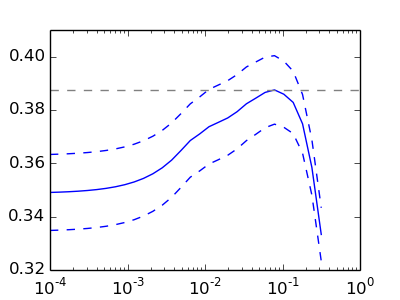This documentation is for scikit-learn version 0.15-gitOther versions

If you use the software, please consider citing scikit-learn.

# Cross-validation on diabetes Dataset Exercise¶

A tutorial excercise which uses cross-validation with linear models.

This exercise is used in the Cross-validated estimators part of the Model selection: choosing estimators and their parameters section of the A tutorial on statistical-learning for scientific data processing.Script output:

```Answer to the bonus question: how much can you trust the selection of alpha?

Alpha parameters maximising the generalization score on different
subsets of the data:
[fold 0] alpha: 0.10405, score: 0.53573
[fold 1] alpha: 0.05968, score: 0.16278
[fold 2] alpha: 0.10405, score: 0.44437

Answer: Not very much since we obtained different alphas for different
subsets of the data and moreover, the scores for these alphas differ
quite substantially.
```

Python source code: plot_cv_diabetes.py

```from __future__ import print_function
print(__doc__)

import numpy as np
import matplotlib.pyplot as plt

from sklearn import cross_validation, datasets, linear_model

X = diabetes.data[:150]
y = diabetes.target[:150]

lasso = linear_model.Lasso()
alphas = np.logspace(-4, -.5, 30)

scores = list()
scores_std = list()

for alpha in alphas:
lasso.alpha = alpha
this_scores = cross_validation.cross_val_score(lasso, X, y, n_jobs=1)
scores.append(np.mean(this_scores))
scores_std.append(np.std(this_scores))

plt.figure(figsize=(4, 3))
plt.semilogx(alphas, scores)
# plot error lines showing +/- std. errors of the scores
plt.semilogx(alphas, np.array(scores) + np.array(scores_std) / np.sqrt(len(X)),
'b--')
plt.semilogx(alphas, np.array(scores) - np.array(scores_std) / np.sqrt(len(X)),
'b--')
plt.ylabel('CV score')
plt.xlabel('alpha')
plt.axhline(np.max(scores), linestyle='--', color='.5')

##############################################################################
# Bonus: how much can you trust the selection of alpha?

# To answer this question we use the LassoCV object that sets its alpha
# parameter automatically from the data by internal cross-validation (i.e. it
# performs cross-validation on the training data it receives).
# We use external cross-validation to see how much the automatically obtained
# alphas differ across different cross-validation folds.
lasso_cv = linear_model.LassoCV(alphas=alphas)
k_fold = cross_validation.KFold(len(X), 3)

"how much can you trust the selection of alpha?")
print()
print("Alpha parameters maximising the generalization score on different")
print("subsets of the data:")
for k, (train, test) in enumerate(k_fold):
lasso_cv.fit(X[train], y[train])
print("[fold {0}] alpha: {1:.5f}, score: {2:.5f}".
format(k, lasso_cv.alpha_, lasso_cv.score(X[test], y[test])))
print()
print("Answer: Not very much since we obtained different alphas for different")
print("subsets of the data and moreover, the scores for these alphas differ")
print("quite substantially.")

plt.show()
```

Total running time of the example: 0.27 seconds ( 0 minutes 0.27 seconds)